# The OPTQP Procedure

### PROC OPTQP Statement

The following options can be specified in the PROC OPTQP statement.

DATA=SAS-data-set

specifies the input SAS data set. This data set can also be created from a QPS-format flat file by using the SAS macro %MPS2SASD. If the DATA= option is not specified, PROC OPTQP uses the most recently created SAS data set. See Chapter 15: The MPS-Format SAS Data Set, for more details.

DUALOUT=SAS-data-set
DOUT=SAS-data-set

specifies the output data set to contain the dual solution. See the section Output Data Sets for details.

LOGFREQ=k
PRINTFREQ=k

specifies that the printing of the solution progress to the iteration log should occur after every k iterations. The print frequency, k, is an integer between zero and the largest four-byte, signed integer, which is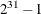. The value k = 0 disables the printing of the progress of the solution. The default value of this option is 1.

MAXITER=k

specifies the maximum number of predictor-corrector iterations performed by the interior point algorithm (see the section Interior Point Algorithm: Overview). The value k is an integer between 1 and the largest four-byte, signed integer, which is. If you do not specify this option, the procedure does not stop based on the number of iterations performed.

MAXTIME=t

specifies an upper limit ofseconds of time for reading in the data and performing the optimization process. The value of the TIMETYPE= option determines the type of units used. If you do not specify this option, the procedure does not stop based on the amount of time elapsed. The value of t can be any positive number; the default value is the positive number that has the largest absolute value that can be represented in your operating environment.

OBJSENSE=option

specifies whether the QP model is a minimization or a maximization problem. You specify OBJSENSE=MIN for a minimization problem and OBJSENSE=MAX for a maximization problem. Alternatively, you can specify the objective sense in the input data set; see the section ROWS Section for details. If the objective sense is specified differently in these two places, this option supersedes the objective sense specified in the input data set. If the objective sense is not specified anywhere, then PROC OPTQP interprets and solves the quadratic program as a minimization problem.

PRESOLVER=number | string
PRESOL=number | string

specifies one of the following presolve options:

number

string

Description

0

NONE

Disables the presolver.

–1

AUTOMATIC

Applies the presolver by using default setting.

1

BASIC

Applies the basic presolver.

2

MODERATE

Applies the moderate presolver.

3

AGGRESSIVE

Applies the aggressive presolver.

You can specify the option either by a word or by integers from –1 to 3. The default option is AUTOMATIC.

PRIMALOUT=SAS-data-set
POUT=SAS-data-set

specifies the output data set to contain the primal solution. See the section Output Data Sets for details.

PRINTLEVEL=01

specifies whether a summary of the problem and solution should be printed. If PRINTLEVEL=1, then the Output Delivery System (ODS) tables ProblemSummary, SolutionSummary, and PerformanceInfo are produced and printed. If PRINTLEVEL=2, then the same tables are produced and printed along with an additional table called ProblemStatistics. If PRINTLEVEL=0, then no ODS tables are produced or printed. The default value is 1.

For details about the ODS tables created by PROC OPTQP, see the section ODS Tables.

SAVE_ONLY_IF_OPTIMAL

specifies that the PRIMALOUT= and DUALOUT= data sets be saved only if the final solution obtained by the solver at termination is optimal. If the PRIMALOUT= or DUALOUT= option is specified, and this option is not specified, then the output data sets will only contain solution values at optimality. If the SAVE_ONLY_IF_OPTIMAL option is not specified, the output data sets will not contain an intermediate solution.

STOP_DG=
specifies the desired relative duality gap,
[1E–9, 1E–4]. This is the relative difference between the primal and dual objective function values and is the primary solution quality parameter. The default value is 1E–6. See the section Interior Point Algorithm: Overview for details.

STOP_DI=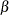specifies the maximum allowed relative dual constraints violation,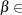[1E–9, 1E–4]. The default value is 1E–6. See the section Interior Point Algorithm: Overview for details.

STOP_PI=specifies the maximum allowed relative bound and primal constraints violation,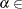[1E–9, 1E–4]. The default value is 1E–6. See the section Interior Point Algorithm: Overview for details.

TIMETYPE=number | string

specifies whether CPU time or real time is used for the MAXTIME= option and the _OROPTQP_ macro variable in a PROC OPTQP call. Table 12.2 describes the valid values of the TIMETYPE= option.

Table 12.2: Values for TIMETYPE= Option

number

string

Description

0

CPU

Specifies units of CPU time.

1

REAL

Specifies units of real time.

The default value of the TIMETYPE= option depends on the value of the NTHREADS= option in the PERFORMANCE statement. See the section PERFORMANCE Statement for more information about the NTHREADS= option.

If you specify a value greater than 1 for the NTHREADS= option, the default value of the TIMETYPE= option is REAL. If you specify a value of 1 for the NTHREADS= option, the default value of the TIMETYPE= option is CPU.